# Navier-Stokes equations

## Continuity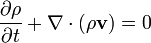$\frac{\partial \rho}{\partial t} + \nabla \cdot (\rho \mathbf{v}) = 0$

or, using the substantive derivative: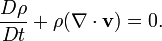$\frac{D\rho}{Dt} + \rho (\nabla \cdot \mathbf{v}) = 0.$

For an incompressible fluid,$\rho$ is constant, hence the velocity field must be divergence-free: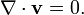$\nabla \cdot \mathbf{v} =0.$

## Momentum

(Also known as the Navier-Stokes equation.)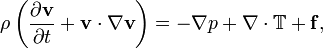$\rho \left(\frac{\partial \mathbf{v}}{\partial t} + \mathbf{v} \cdot \nabla \mathbf{v} \right) = -\nabla p + \nabla \cdot\mathbb{T} + \mathbf{f},$

or, using the substantive derivative: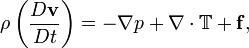$\rho \left(\frac{D \mathbf{v}}{D t} \right) = -\nabla p + \nabla \cdot\mathbb{T} + \mathbf{f},$

where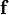$\mathbf{f}$ is a volumetric force (e.g.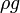$\rho g$ for gravity), and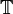$\mathbb{T}$ is the stress tensor.

## Stress

The vector quantity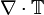$\nabla \cdot\mathbb{T}$ is the shear stress. For a Newtonian incompressible fluid,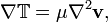$\nabla \mathbb{T} = \mu \nabla^2 \mathbf{v},$

with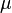$\mu$ being the (dynamic) viscosity.

For an inviscid fluid, the momentum equation becomes Euler's equation for ideal fluids: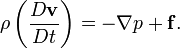$\rho \left(\frac{D \mathbf{v}}{D t} \right) = -\nabla p + \mathbf{f} .$